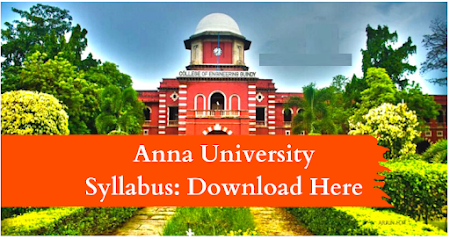### EC8553   DISCRETE-TIME SIGNAL PROCESSING                      L T P C 4 0 0 4

OBJECTIVES:  To learn discrete fourier transform, properties of DFT and its application to linear filtering  To understand the characteristics of digital filters, design digital IIR and FIR filters and apply these filters to filter undesirable signals in various frequency bands  To understand the effects of finite precision representation on digital filters  To understand the fundamental concepts of multi rate signal processing and its applications  To introduce the concepts of adaptive filters and its application to communication engineering

UNIT I DISCRETE FOURIER TRANSFORM                                                                             12 Review of signals and systems, concept of frequency in discrete-time signals, summary of analysis & synthesis equations for FT & DTFT, frequency domain sampling, Discrete Fourier transform (DFT) - deriving DFT from DTFT, properties of DFT - periodicity, symmetry, circular convolution. Linear filtering using DFT. Filtering long data sequences - overlap save and overlap add method. Fast computation of DFT - Radix-2 Decimation-in-time (DIT) Fast Fourier transform (FFT), Decimation-in-frequency (DIF) Fast Fourier transform (FFT). Linear filtering using FFT.

UNIT II INFINITE IMPULSE RESPONSE FILTERS                                                                 12 Characteristics of practical frequency selective filters. characteristics of commonly used analog filters - Butterworth filters, Chebyshev filters. Design of IIR filters from analog filters (LPF, HPF, BPF, BRF) - Approximation of derivatives, Impulse invariance method, Bilinear transformation. Frequency transformation in the analog domain. Structure of IIR filter - direct form I, direct form II, Cascade, parallel realizations.

UNIT III FINITE IMPULSE RESPONSE FILTERS                                                                12
Design of FIR filters - symmetric and Anti-symmetric FIR filters - design of linear phase FIR filters using Fourier series method - FIR filter design using windows (Rectangular, Hamming and Hanning window), Frequency sampling method. FIR filter structures - linear phase structure, direct form realizations

UNIT IV FINITE WORD LENGTH EFFECTS                                                                          12 Fixed point and floating point number representation - ADC - quantization - truncation and rounding - quantization noise - input / output quantization - coefficient quantization error - product quantization error - overflow error - limit cycle oscillations due to product quantization and summation - scaling to prevent overflow.

UNIT V INTRODUCTION TO DIGITAL SIGNAL PROCESSORS                                        12 DSP functionalities - circular buffering – DSP architecture – Fixed and Floating point architecture principles – Programming – Application examples.

TOTAL:60 PERIODS

OUTCOMES: At the end of the course, the student should be able to  Apply DFT for the analysis of digital signals and systems  Design IIR and FIR filters  Characterize the effects of finite precision representation on digital filters  Design multirate filters  Apply adaptive filters appropriately in communication systems

TEXT BOOK:
1. John G. Proakis & Dimitris G.Manolakis, ―Digital Signal Processing – Principles, Algorithms & Applications‖, Fourth Edition, Pearson Education / Prentice Hall, 2007. (UNIT I – V)

REFERENCES:
1. Emmanuel C. Ifeachor & Barrie. W. Jervis, ―Digital Signal Processing‖, Second Edition, Pearson Education / Prentice Hall, 2002.
2. A. V. Oppenheim, R.W. Schafer and J.R. Buck, ―Discrete-Time Signal Processing‖, 8th Indian Reprint, Pearson, 2004.
3. Sanjit K. Mitra, ―Digital Signal Processing – A Computer Based Approach‖, Tata Mc Graw Hill, 2007.
4. Andreas Antoniou, ―Digital Signal Processing‖, Tata Mc Graw Hill, 2006.